Matrices
 Home > Lessons > Matrices Search | Updated April 11th, 2019
Introduction

In this section, you will learn how to calculate the equation of a line using the point-slope formula. Here are the sections within this lesson:

Matrices are an organized list of numbers. They look like blocks of numbers, like this matrix, which we will call matrix A.Matrices have certain characteristics. One characteristic is to describe a matrix by its dimensions. To do so, you have to understand that matrices have rows and columns. Rows go left to right.It can be seen that matrix A has three rows. We can also look at a matrix's columns, which go up and down. Matrix A has two columns.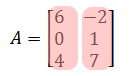When we describe a matrix by its dimensions, we report its number of rows first, then the number of columns. Matrix A is therefore a '3 by 2' matrix, which is written as '3x2.'A 2x3 matrix is shaped much differently, like matrix B.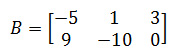Matrix B has 2 rows and 3 columns.

We call numbers or values within the matrix 'elements.' There are six elements in both matrix A and matrix B. Here is matrix C. What are its dimensions and how many elements does it have?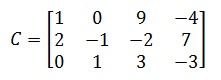Matrix C is a 3x4 matrix and it has 12 elements. In the 2nd row and the 3rd column, the value -2 can be found. In the 1st row, 3rd column, the value 9 can be found.

When businesses deal with sales, there is a need to organize information. For instance, let's say Karadimos King is a fast-food restaurant that made the following number of sales:

On Monday, Karadimos King sold 35 hamburgers, 50 sodas, and 45 fries. On Tuesday, it sold 120 sodas, 56 fries, and 43 hamburgers. On Wednesday, it sold 15 fries, 12 hamburgers, and 19 sodas.

To keep an inventory of this information, a programmer creates a matrix to hold this information. The information can be used to determine how much profit was made, how many items were used, and which days were most productive for the business.

To place the information into a matrix, someone has to decide how to organize it. We can make columns that represent items and list the items like so: hamburgers, fries, and sodas. Along the rows we can place days of the week, like so.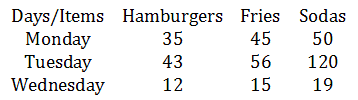Notice how the information was carefully placed into a table of values, making sure hamburgers were the first values, fries were the second, and sodas the third.

This means our new matrix is this 3x3 9-element matrix without the row and column descriptors.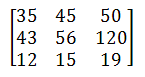Let's say we have Monday's lunch data from our restaurant, Karadimos King, as follows.Let's say we have Tuesday's lunch data from our restaurant, too.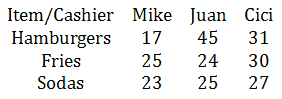If we want to total up the inventory for the two days, we would add the matrices.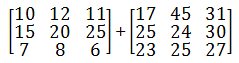Intuitively speaking, it makes sense to add corresponding elements from each matrix, like so.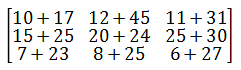Mike's information is being added to his own, by item: hamburgers with hamburgers, fries with fries, ... Likewise, the same is being done with Juan and Cici's sales information. The final result is this.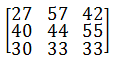This information is a two day total for three cashiers and it's still organized by cashier.

There is a word of caution dealing with matrix addition. Matrices of the exact same dimensions can only be added together. So, these two matrices cannot be added together.The first matrix is a 2x2 matrix and the second is a 1x3 matrix. The two matrices are of different size and therefore cannot logically be combined.

Try this quizmaster to determine if you understand the lesson above.uiz: Adding Matrices

In our last section, we encountered two days of lunch data for the Karadimos King restaurant: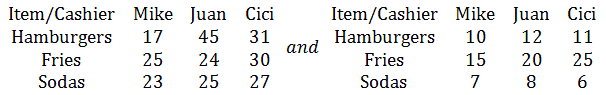I placed Tuesday's data first, because I noticed more items were sold on Tuesday. If we wanted to know how many more items were sold on Tuesday, we would subtract the two matrices like so.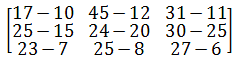Notice how the each corresponding element is subtracted. The result is a matrix that still is organized by item and by cashier.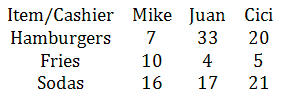This means Mike sold 10 more fries on Tuesday than he did on Monday at Karadimos King. Cici sold 20 more hamburgers on the second day.

Again, a word of caution. Matrices of exactly the same dimensions can only be subtracted. These matrices cannot be subtracted.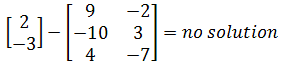The first matrix is a 2x1 and the second is a 3x2. Since their dimensions do not exactly match, they cannot be subtracted.

Try this quizmaster to determine if you understand the lesson above.uiz: Subtracting Matrices

Scalars are quantities. For instance, multiplying a matrix times a number is an example of scalar multiplication.

If we grab a matrix from a previous section, this can be easily explained. Here is Tuesday's sales inventory for three cashiers and three items.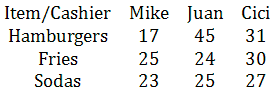If we assume that sales like this will continue in this fashion for a month, we can estimate sales. We can project the inventory we need by multiplying these sales by 30 to determine how many items we will sell for 30 days.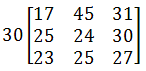Doing so would yield this.Notice how every element within the matrix is multiplied by the scalar quantity, 30. Here is the projected result of the all the items that should be sold if our estimation is accurate.Try this quizmaster to determine if you understand the lesson above.uiz: Matrices: Scalar Multiplication

Multiplying matrices is by far the most tedious of all operations involving matrices. It, quite honestly, involves a number of complicated steps best left for an advanced calculator, like a TI-81 or better. Many other companies, like Casio, make great calculators, too, that can handle matrices, including matrix multiplication.

For the purposes of this lesson on matrices, I'll demonstrate matrix multiplication using two small matrices.

Let's say we needed to take our inventory matrix and determine how much the pre-tax sales will be. This is our Monday inventory for lunch at Karadimos King.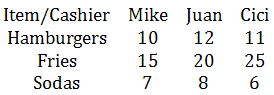Another way to look at the data is to switch the rows and columns. Doing this will help us create matrices that are easier to follow for the purposes of matrix multiplication.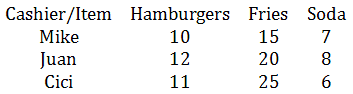The cost of a hamburger is \$2.75. The cost of an order of fries is \$1.50 and the cost of a soda is \$0.90. We can make a column matrix with this information.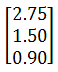Now, we will multiply the two matrices to gain a matrix of customer costs or pre-tax money that will be obtained from the customers.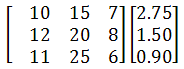To multiply, we take the first row of the first matrix and multiply it by the column of the second matrix, like so.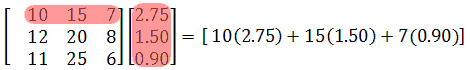The result is 56.30. Since we used row #1 of the first matrix and column #1 from the second matrix, this element goes into the 1st row, 1st column of a new matrix.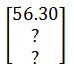We are going to slowly build our answer, which is obviously a new matrix. Moving on, we will grab row #2 from the first matrix and column #1 from the second matrix and multiply.The answer is 70.2. Since we used row #2 of the first matrix and column #1 from the second matrix, this element goes into the 2nd row, 1st column of our new matrix.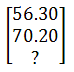We will now grab row #3 from the first matrix and column #1 from the second matrix and multiply.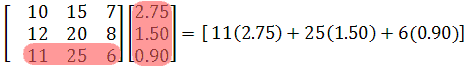The answer is 73.15. Since we used row #3 of the first matrix and column #1 from the second matrix, this element goes into the 3rd row, 1st column of our answer matrix.This is our final solution to our matrix multiplication problem. It indicates how much pre-tax money our cashiers should have collected.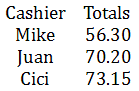Due to the tedious nature of matrix multiplication and the likelihood of making a small calculation error, it is strongly advised that this operation be done using an advanced calculator. However, if you are forced to multiply matrices without assistance, the video below will help you understand how matrix multiplication is done with larger matrices.ideo: Matrix Multiplicationuiz: Multiplying Matrices

Try an activity related to the lessons above.ideo: Matrix Multiplication

Try these quizmasters to test your skills.uiz: Adding Matricesuiz: Subtracting Matricesuiz: Matrices: Scalar Multiplicationuiz: Multiplying Matrices

Try an activity related to the lessons above.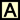ctivity: Matrix Basicsctivity: Matrix Discoveryctivity: Matrix Variety

Try these related lessons.esson: The Inverse of a Matrixesson: The Determinant of a Matrixesson: Matrix Equations